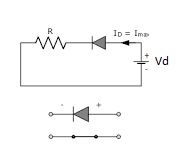What is a Diode? Characteristics and Operation - LBM4

## Diode

Diode is a semi conductor device that produces no current when operated in reversed biased mode and zero voltage drops when operaed in forward biased mode. Thus, a forward biased diode acts as a short circuit and reversed biased diode acts as an open circuit.

## Terminal Characteristics of Junction Diodes

From Shcokley's diode equation, we have,
I_D=I_S\left(e^{V_D/ηV_T}-1\ \right)......\left(i\right)
where,
V_T=\frac{KT}{q}
where,
K=Boltzmann's constatnt
T=absolute temperature in Kelvin
q=magnitude of electronic charge

### Forward Biased mode (with Vd>0)

we have, I_D=I_S\left(e^{V_D/ηV_T}-1\ \right)......\left(i\right)
or, I_{D1}=I_{S\ }e^{V_{D1}/ηV_T}
Also, I_{D2}=I_{S\ }e^{V_{D2}/ηV_T}
Dividing above both equation
\frac{I_{D2}}{I_{D1}}=\ e^{\frac{V_{D2}-V_{D1}}{ηV_T}}
V_{D2}-V_{D1}=\ ηV_T\ln \frac{I_{D2}}{I_{D1}}
V_{D2}-V_{D1}=\ 2.3ηV_T\log \frac{I_{D2}}{I_{D1}}

## Physical Operation of Diode

### No Biasing applied ( Vd=0 V)

In absence of an applied bias, the net flow of electron or holes (charges) in one direction is zero. Hence, no current flows if no biasing voltage is applied.

### Forward Bias Region

A forward bias condition is established by applying the positive potential to the p-type material and the negative potential to n-type material as shown in figure.A glance at the I-V characteristics in the forward region reveals that the current is negligibly small for Vd smaller than about 0.5 V. This value is usually referred as the cut-in voltage. But for a "fully conducting diode" the voltage drop lies in a narrow range, approximately 0.6 V to 0.8 V

### Reverse Bias Region

The reverse bias region of operation is entered when the diode voltage Vd is negative.
We have,
I_D=I_S\left(e^{V_D/ηV_T}-1\ \right)......\left(i\right)
In equation (i) if Vd is negative and a few times larger than Vt (25mV) in magnitude, the exponential term becomes negligbly small compared to unity, and the diode currents becomes
I_D=I_S

### Breakdown Region

The breakdown region can be easily identified on the diode I-V characteristics. The breakdown region is entered when the magnitude of the reverse voltage exceeds a threshold voltage that is specific to the particular diode, is called the "breakdown voltage". This voltage is at the"knee" of the I-V curve and is denoted Vzk.